# Surface Area

## Calculate the surface areas of the given basic solid shapes using standard formulae.

##### MenuLevel 1Level 2Level 3Level 4Level 5Level 6Level 7Level 8Level 9ExamHelp

This is level 1; Find the surface area of shapes made up of cubes. The diagrams are not to scale.

 1 Each of the yellow cubes in the diagram have edges 1cm long.What is the total surface area of the cuboid they are part of?☐ cm2 ☐ ✓ ✗ 2 Each of the yellow cubes in the diagram have edges 1cm long.What is the total surface area of the shape they are part of?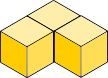☐ cm2 ☐ ✓ ✗ 3 Each of the yellow cubes in the diagram have edges 1cm long.What is the total surface area of the shape they are part of?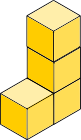☐ cm2 ☐ ✓ ✗ 4 Each of the yellow cubes in the diagram have edges 1cm long.What is the total surface area of the shape they are part of?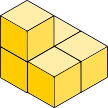☐ cm2 ☐ ✓ ✗ 5 Each of the cubes in the diagram have edges 2cm long.What is the total surface area of the shape they are part of?☐ cm2 ☐ ✓ ✗ 6 Each of the cubes in the diagram have edges 3cm long.What is the total surface area of the shape they are part of?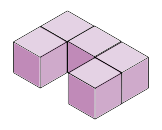☐ cm2 ☐ ✓ ✗
Check

This is Surface Area level 1. You can also try:
Level 2 Level 3 Level 4 Level 5 Level 6 Level 7 Level 8 Level 9

## Instructions

Try your best to answer the questions above. Type your answers into the boxes provided leaving no spaces. As you work through the exercise regularly click the "check" button. If you have any wrong answers, do your best to do corrections but if there is anything you don't understand, please ask your teacher for help.

When you have got all of the questions correct you may want to print out this page and paste it into your exercise book. If you keep your work in an ePortfolio you could take a screen shot of your answers and paste that into your Maths file.

## Transum.org

This web site contains over a thousand free mathematical activities for teachers and pupils. Click here to go to the main page which links to all of the resources available.## More Activities:

Mathematicians are not the people who find Maths easy; they are the people who enjoy how mystifying, puzzling and hard it is. Are you a mathematician?

Comment recorded on the 23 September 'Starter of the Day' page by Judy, Chatsmore CHS:

"This triangle starter is excellent. I have used it with all of my ks3 and ks4 classes and they are all totally focused when counting the triangles."

Comment recorded on the 3 October 'Starter of the Day' page by Mrs Johnstone, 7Je:

"I think this is a brilliant website as all the students enjoy doing the puzzles and it is a brilliant way to start a lesson."

Each month a newsletter is published containing details of the new additions to the Transum website and a new puzzle of the month.

The newsletter is then duplicated as a podcast which is available on the major delivery networks. You can listen to the podcast while you are commuting, exercising or relaxing.

Transum breaking news is available on Twitter @Transum and if that's not enough there is also a Transum Facebook page.

#### Lemon Law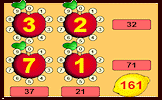A fascinating digit changing challenge. Change the numbers on the apples so that the number on the lemon is the given total. Can you figure out, by understanding place value, how this works?

There are answers to this exercise but they are available in this space to teachers, tutors and parents who have logged in to their Transum subscription on this computer.

A Transum subscription unlocks the answers to the online exercises, quizzes and puzzles. It also provides the teacher with access to quality external links on each of the Transum Topic pages and the facility to add to the collection themselves.

Subscribers can manage class lists, lesson plans and assessment data in the Class Admin application and have access to reports of the Transum Trophies earned by class members.

Subscribe

## Go Maths

Learning and understanding Mathematics, at every level, requires learner engagement. Mathematics is not a spectator sport. Sometimes traditional teaching fails to actively involve students. One way to address the problem is through the use of interactive activities and this web site provides many of those. The Go Maths page is an alphabetical list of free activities designed for students in Secondary/High school.

## Maths Map

Are you looking for something specific? An exercise to supplement the topic you are studying at school at the moment perhaps. Navigate using our Maths Map to find exercises, puzzles and Maths lesson starters grouped by topic.

## Teachers

If you found this activity useful don't forget to record it in your scheme of work or learning management system. The short URL, ready to be copied and pasted, is as follows:

Alternatively, if you use Google Classroom, all you have to do is click on the green icon below in order to add this activity to one of your classes.

It may be worth remembering that if Transum.org should go offline for whatever reason, there is a mirror site at Transum.info that contains most of the resources that are available here on Transum.org.

When planning to use technology in your lesson always have a plan B!

Do you have any comments? It is always useful to receive feedback and helps make this free resource even more useful for those learning Mathematics anywhere in the world. Click here to enter your comments.For All:

Scan the QR code below to visit the online version of this activity.https://Transum.org/go/?Num=264

## Description of Levels

Close

Level 1 - Find the surface area of shapes made up of cubes.

Level 2 - Find the surface area of a variety of cuboids.

Level 3 - Find the surface area of a variety of prisms.

Level 4 - Find the surface area of a variety of cylinders.

Level 5 - Find the surface area of a variety of cones.

Level 6 - Find the surface area of a variety of pyramids.

Level 7 - Find the surface area of a variety of spheres.

Level 8 - Find the surface area of composite shapes.

Level 9 - Mixed, more challenging questions involving surface area.

Volume - Find the volume of basic solid shapes.

Surface Area = Volume - Can you find the ten cuboids that have numerically equal volumes and surface areas? A challenge in using technology.

Exam Style Questions - A collection of problems in the style of GCSE or IB/A-level exam paper questions (worked solutions are available for Transum subscribers).

More on 3D Shapes including lesson Starters, visual aids, investigations and self-marking exercises.

Answers to this exercise are available lower down this page when you are logged in to your Transum account. If you don’t yet have a Transum subscription one can be very quickly set up if you are a teacher, tutor or parent.

## Surface Area Formulae

Cube: $$6s^2$$ where $$s$$ is the length of one edge.

Cuboid: $$2(lw + lh + wh)$$ where $$l$$ is the length, $$w$$ is the width and $$h$$ is the height of the cuboid.

Cylinder: $$2\pi rh + 2\pi r^2$$ where $$h$$ is the height (or length) of the cylinder and $$r$$ is the radius of the circular end.

Cone: $$\pi r(r+l)$$ where $$l$$ is the distance from the apex to the rim of the circle (sloping height) of the cone and $$r$$ is the radius of the circular base.

Cone: $$\pi r(r+\sqrt{h^2+r^2})$$ where $$h$$ is the height of the cone and $$r$$ is the radius of the circular base.

Square based pyramid: $$s^2+2s\sqrt{\frac{s^2}{4}+h^2}$$ where $$h$$ is the height of the pyramid and $$s$$ is the length of a side of the square base.

Rectangular based pyramid: $$lw+l\sqrt{\frac{w^2}{4}+h^2}+w\sqrt{\frac{l^2}{4}+h^2}$$ where $$h$$ is the height of the pyramid, $$l$$ is the length of the base and $$w$$ is the width of the base.

Sphere: $$4\pi r^2$$ where $$r$$ is the radius of the sphere.

Prism: Double the area of the cross section added to the product of the length and the perimeter of the cross section.

Answers to this exercise are available lower down this page when you are logged in to your Transum account. If you don’t yet have a Transum subscription one can be very quickly set up if you are a teacher, tutor or parent.

Close